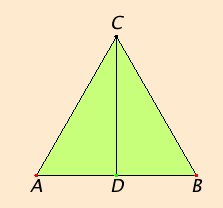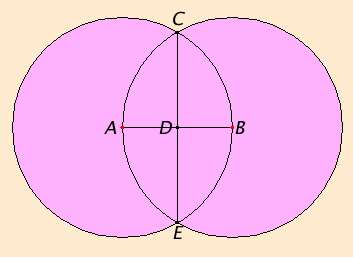# Proposition 10

To bisect a given finite straight line.

Let AB be the given finite straight line.It is required to bisect the finite straight line AB.

Construct the equilateral triangle ABC on it, and bisect the angle ACB by the straight line CD.

I say that the straight line AB is bisected at the point D.

Since CA equals CB, and CD is common, therefore the two sides CA and CD equal the two sides CB and CD respectively, and the angle ACD equals the angle BCD, therefore the base AD equals the base BD.

Therefore the given straight line AB is bisected at D.

Q.E.F.

## Guide

While this construction divides a line into two equal parts, the construction in proposition VI.9 divides a line into any given number of equal parts.

#### Construction steps

This method for bisecting lines takes less actual work than it appears to. It is really no more than the double-equilateral-triangle.

 First, the equilateral triangle ABC needs to be constructed. According to I.1 two circles need to be drawn: one with center A and radius AB, the other with center B and radius BA. One of the points of intersection of the two circles is C. Then to bisect angle ACB, according to I.9, an arbitrary point is chosen on one side of the angle, and it might as well be the point A on the side AC, and a point equally far from C on the side BC, which is, of course, B. Then an equilateral triangle is constructed on the line AB. There are two such equilateral triangles, the one already constructed ACB, and another one, call it AEB. The point E is the other intersection of the two circles already drawn. Then, by I.9, the line CE bisects the angle ACB, and according to this proposition, the point D bisects the line AB. Actually, only two circles and the straight line CE need to be drawn. The straight lines AC, CB, AE, and EB, aren’t necessary for the construction; they are only used to show that the construction is correct.#### Use of Proposition 10

The construction of this proposition in Book I is used in propositions I.12, I.16, and I.42. It is also used in several propositions in the Books II, III, IV, X, and XIII.